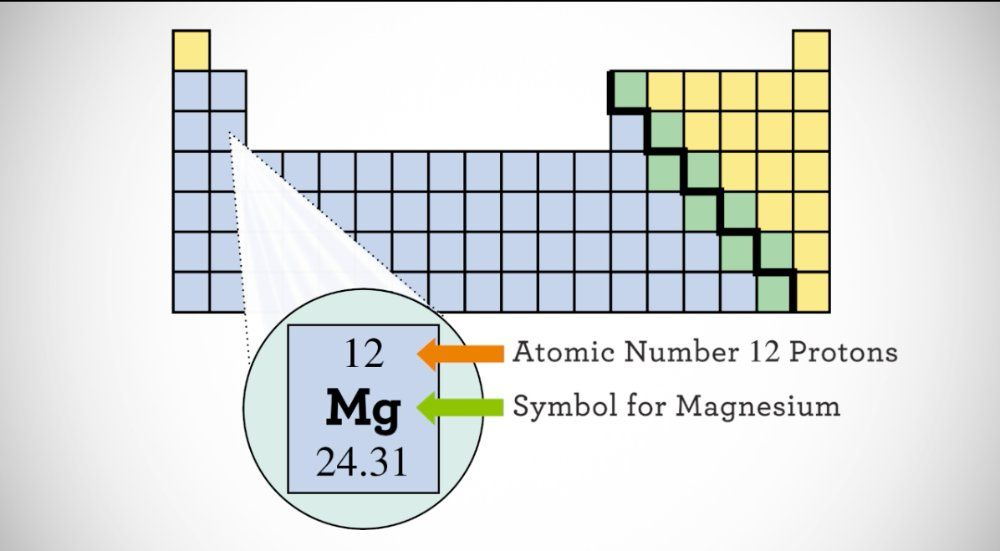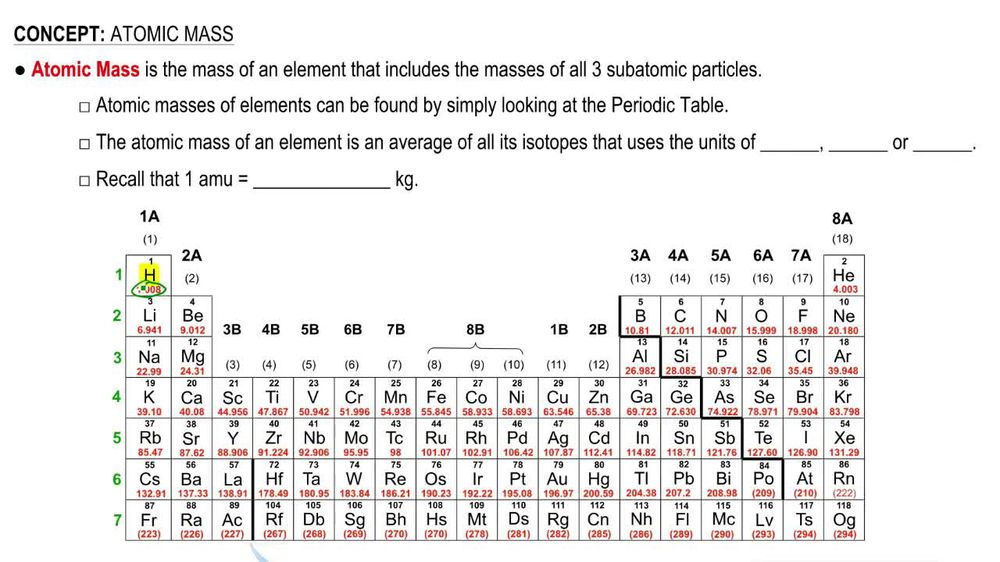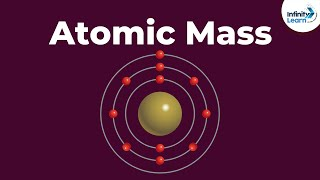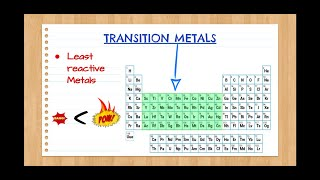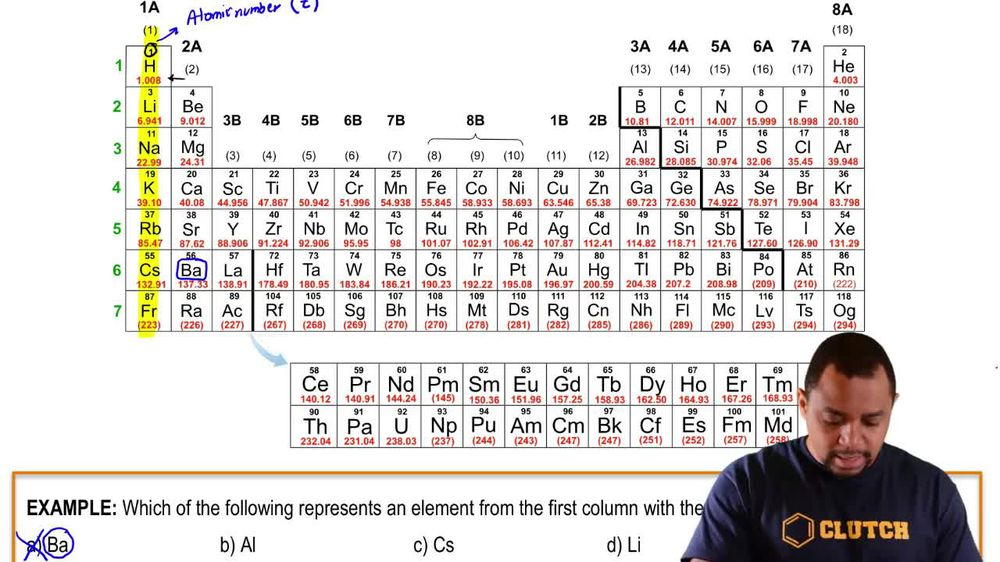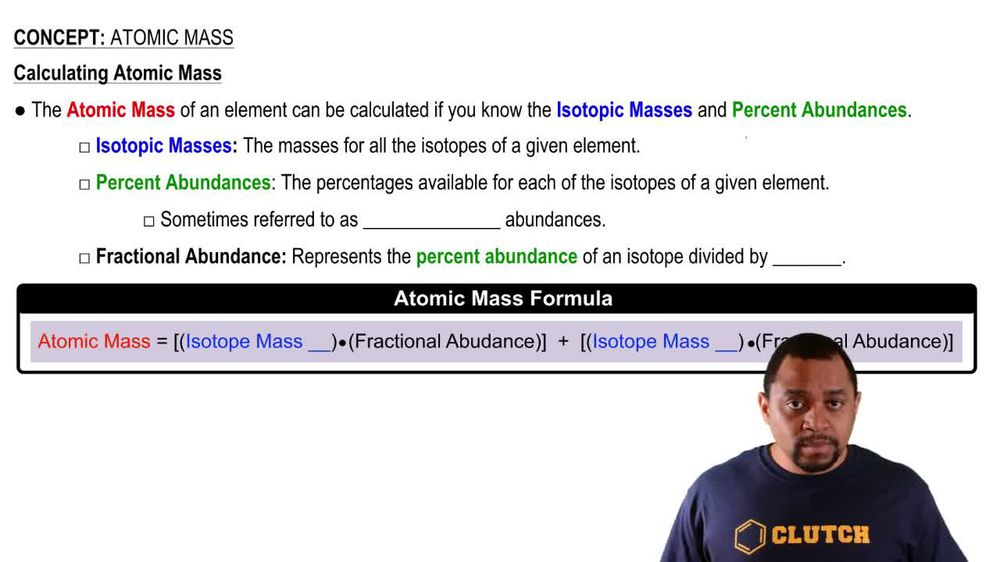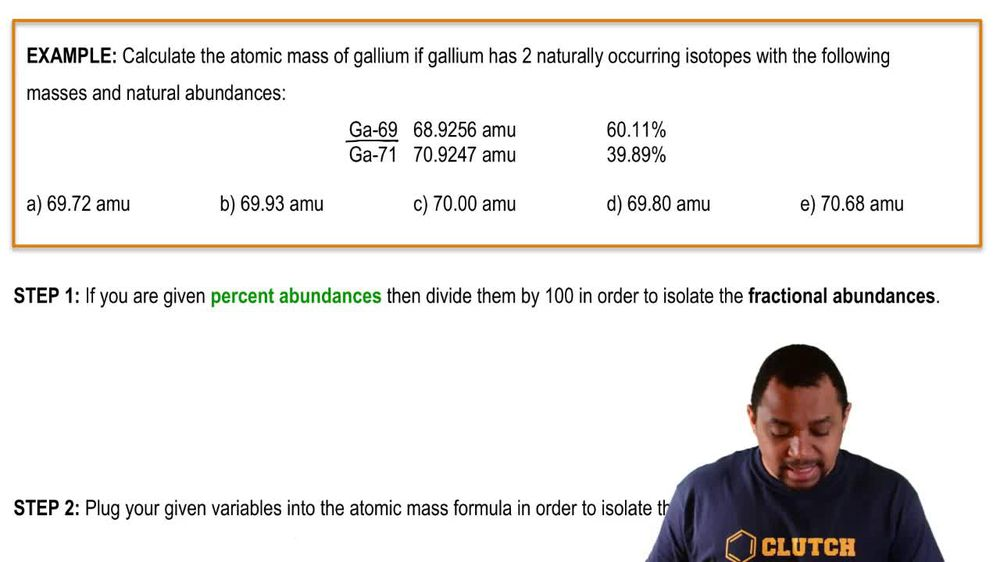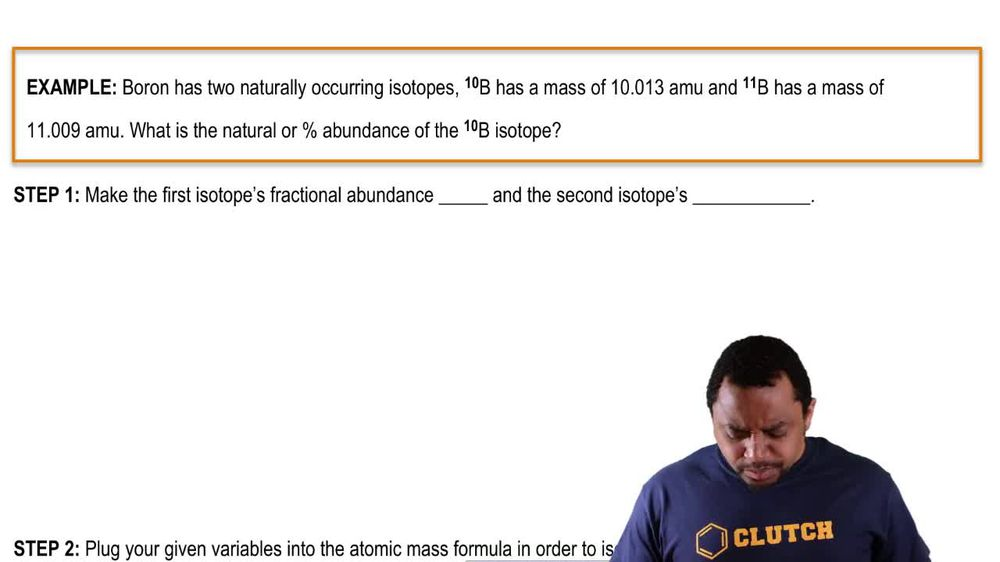Start typing, then use the up and down arrows to select an option from the list.
1. 2. Atoms & Elements2. Atomic Mass
Problem

# Germanium has five naturally occurring isotopes: 70^Ge, 20.5%, 69.924; 72^Ge, 27.4%, 71.922; 73^Ge, 7.8%, 72.923; 74^Ge, 36.5%, 73.921; and 76^Ge, 7.8%, 75.921. What is the atomic weight of germanium?

Relevant Solution2m
Play a video:
Hi everyone for this problem, it reads the natural isotopes of sulfur are listed below, calculate the atomic weight of sulfur. Okay. So in order for us to calculate the atomic weight were given everything that we need in this problem because atomic weight is the weighted average of the mass of natural isotopes by their abundance. So we know the weighted average of the masses and we know their abundance. So we're going to take the sum of each. Okay? Or we're going to take the sum for all of them. So atomic weight Is equal to so we know we have four Of the natural isotopes. So for the first one, so for 32 its abundance, we're going to convert this to decimal form. So its abundance at 0. 99. And we're going to multiply it by its atomic mass unit. So 31.972. Okay, so this is the first isotope. The second isotope is abundance is 0.0075. We're taking the percent and converting them all to decimals. Okay, So .0075 times its atomic mass unit. 32.971. Okay, our third isotope, so for 34 its abundance is 0.04-5. And its atomic mass unit is 33.968. And our last isotope. So for 36 its abundance is 0.001 And its atomic mass unit is 35.967. Okay, so that gives us once we take the some The atomic weight is equal to 32.065 atomic mass units. Okay, so this is going to be our final answer. And what we did was we took the weighted average of the mass of each natural isotope by their abundance. And this is our final answer. That's it for this problem. I hope this was helpful.